# Number of divisors

(diff) ← Older revision | Latest revision (diff) | Newer revision → (diff)

A function of a natural argument,, equal to the number of natural divisors of the number. This arithmetic function is denoted byor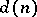. The following formula holds: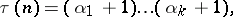where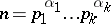is the canonical expansion ofinto prime factors. For prime numbers,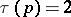, but there exists an infinite sequence offor which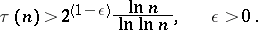On the other hand, for all,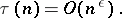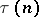is a multiplicative arithmetic function and is equal to the number of points with natural coordinates on the hyperbola. The average value ofis given by Dirichlet's asymptotic formula (cf. Divisor problems). The function, which is the number of solutions of the equationin natural numbers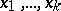, is a generalization of the function.

How to Cite This Entry:
Number of divisors. Encyclopedia of Mathematics. URL: http://encyclopediaofmath.org/index.php?title=Number_of_divisors&oldid=18293
This article was adapted from an original article by N.I. Klimov (originator), which appeared in Encyclopedia of Mathematics - ISBN 1402006098. See original article